### Example 13.5 Resource Allocation Problem

This example describes a model for selecting tasks to be run on a shared resource (Gamrath, 2010). Consider a setof tasks and a resource capacity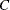. Each item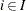has a profit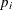, a resource utilization level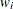, a starting period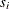, and an ending period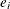. The time horizon considered is from the earliest starting time to the latest ending time of all tasks. With each task, associate a binary variable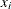, which, if set to, indicates that the task is running from its start time until just before its end time. A task consumes capacity if it is running. The goal is to select which tasks to run in order to maximize profit while not exceeding the shared resource capacity. Let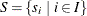define the set of start times for all tasks, and let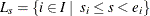define the set of tasks that are running at each start time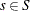. The problem can be modeled as a mixed integer linear programming problem as follows: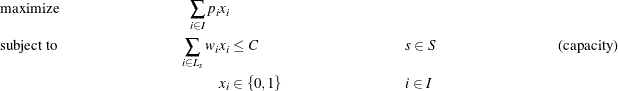In this formulation, constraints (capacity) ensure that the running tasks do not exceed the resource capacity. To illustrate, consider the following five-task example with data: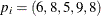,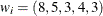,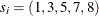,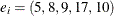, and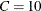. The formulation leads to a constraint matrix that has a staircase structure that is determined by tasks coming on and offline: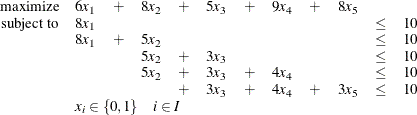#### Lagrangian Decomposition

This formulation clearly has no decomposable structure. However, you can use a common modeling technique known as Lagrangian decomposition to bring the model into block-angular form. Lagrangian decomposition works by first partitioning the constraints into blocks. Then, each original variable is split into multiple copies of itself, one copy for each block in which the variable has a nonzero coefficient in the constraint matrix. Constraints are added to enforce the equality of each copy of the original variable. Then, the original constraints can be written in block-angular form by using the duplicate variables.

To apply Lagrangian decomposition to the resource allocation problem, define a set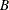of blocks and let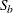define the set of start times for a given block, such that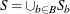. Given this partition of start times, let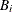define the set of blocks in which taskis scheduled to be running. Now, for each task, define duplicate variables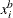for each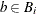. Let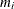define the minimum block index for each class of variable that represents task. The problem can now be modeled in block-angular form as follows: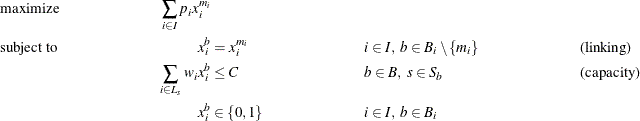In this formulation, constraints (linking) ensure that the duplicate variables are equal to the original variables. Now, the five-task example has been transformed from a staircase structure to a block-angular structure: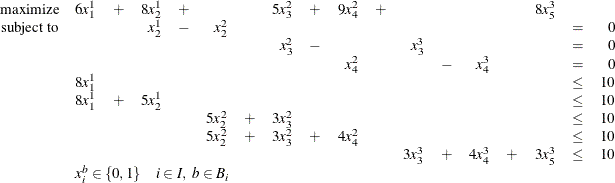To show how to apply Lagrangian decomposition in PROC OPTMODEL, consider the following data set TaskData from Caprara, Furini, and Malaguti (2010) which consists of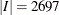tasks:

data TaskData;
input profit weight start end;
datalines;
100 74    1   12
98 32    1    9
73 27    1   22
98 51    1   31
...
23 40 2684 2689
36 85 2685 2687
65 44 2686 2689
18 36 2687 2689
88 57 2688 2689
;


#### Using the MILP Solver Directly in PROC OPTMODEL

The following PROC OPTMODEL statements read in the data and solve the original staircase formulation by calling the MILP solver directly:

%macro SetupData(task_data=, capacity=);
num capacity=&capacity;

/* the set of start times */

set STARTS = setof{i in TASKS} start[i];
/* the set of tasks i that are active at a given start time s */
set TASKS_START{s in STARTS}
= {i in TASKS: start[i] <= s < end[i]};
%mend SetupData;

proc optmodel;

/* select task i to come online from period [start to end) */

/* maximize the total profit of running tasks */
max TotalProfit = sum{i in TASKS} profit[i] * x[i];

/* enforce that the shared resource capacity is not exceeded */
con CapacityCon{s in STARTS}:
sum{i in TASKS_START[s]} weight[i] * x[i] <= capacity;

solve;
quit;
%mend ResourceAllocation_Direct;



The problem summary and solution summary are displayed in Output 13.5.1.

Output 13.5.1: Problem Summary and Solution Summary

The OPTMODEL Procedure

Problem Summary
Objective Sense Maximization
Objective Function TotalProfit
Objective Type Linear

Number of Variables 2697
Bounded Above 0
Bounded Below 0
Bounded Below and Above 2697
Free 0
Fixed 0
Binary 2697
Integer 0

Number of Constraints 2688
Linear LE (<=) 2688
Linear EQ (=) 0
Linear GE (>=) 0
Linear Range 0

Constraint Coefficients 26880

Solution Summary
Solver MILP
Algorithm Branch and Cut
Objective Function TotalProfit
Solution Status Optimal within Relative Gap
Objective Value 62523.999618

Relative Gap 1.5493144E-8
Absolute Gap 0.0009686933
Primal Infeasibility 0
Bound Infeasibility 1.776357E-15
Integer Infeasibility 7.3474993E-6

Best Bound 62524.000587
Nodes 441
Iterations 26866
Presolve Time 1.53
Solution Time 261.86

The iteration log, which contains the problem statistics, the progress of the solution, and the optimal objective value, is shown in Output 13.5.2.

Output 13.5.2: Log

 NOTE: There were 2697 observations read from the data set WORK.TASKDATA. NOTE: Problem generation will use 4 threads. NOTE: The problem has 2697 variables (0 free, 0 fixed). NOTE: The problem has 2697 binary and 0 integer variables. NOTE: The problem has 2688 linear constraints (2688 LE, 0 EQ, 0 GE, 0 range). NOTE: The problem has 26880 linear constraint coefficients. NOTE: The problem has 0 nonlinear constraints (0 LE, 0 EQ, 0 GE, 0 range). NOTE: The OPTMODEL presolver is disabled for linear problems. NOTE: The MILP presolver value AUTOMATIC is applied. NOTE: The MILP presolver removed 0 variables and 0 constraints. NOTE: The MILP presolver removed 0 constraint coefficients. NOTE: The MILP presolver modified 0 constraint coefficients. NOTE: The presolved problem has 2697 variables, 2688 constraints, and 26880 constraint coefficients. NOTE: The MILP solver is called. Node  Active    Sols    BestInteger      BestBound      Gap    Time 0       1       3  54182.0000000         145710   62.82%       2 0       1       3  54182.0000000  73230.2096818   26.01%       2 0       1       6  60619.0000000  70135.8936905   13.57%       3 0       1       7  61216.0000000  68409.0687245   10.51%       4 0       1       7  61216.0000000  67068.0202769    8.73%       5 0       1       7  61216.0000000  66038.0918000    7.30%       6 0       1       7  61216.0000000  65190.7829592    6.10%       7 0       1       7  61216.0000000  64529.6958613    5.14%       8 0       1       7  61216.0000000  64125.0294474    4.54%       9 0       1       7  61216.0000000  63815.0136796    4.07%      10 0       1       7  61216.0000000  63608.2185226    3.76%      10 0       1       7  61216.0000000  63404.4123225    3.45%      10 0       1       7  61216.0000000  63294.7183240    3.28%      11 0       1       7  61216.0000000  63177.6963133    3.11%      11 0       1       7  61216.0000000  63113.0842876    3.01%      11 0       1       7  61216.0000000  63066.7776464    2.93%      11 0       1       7  61216.0000000  62999.6013180    2.83%      11 0       1       7  61216.0000000  62956.5482043    2.76%      11 0       1       7  61216.0000000  62927.3931008    2.72%      11 0       1       7  61216.0000000  62910.5094398    2.69%      12 0       1       7  61216.0000000  62896.8720091    2.67%      12 0       1       7  61216.0000000  62881.4558122    2.65%      12 0       1       7  61216.0000000  62865.7212258    2.62%      12 0       1       8  61282.0000000  62865.7212258    2.52%      12 0       1       8  61282.0000000  62865.7212258    2.52%      12 NOTE: The MILP solver added 1257 cuts with 8396 cut coefficients at the root. 63      61      12  62424.0000000  62814.4025787    0.62%      47 100      97      12  62424.0000000  62807.4025787    0.61%      65 161     151      13  62446.0000000  62758.4665642    0.50%      99 200     187      13  62446.0000000  62739.3314203    0.47%     112 243     175      15  62465.0000000  62718.8175688    0.40%     130 251     124      17  62493.0000000  62717.7127884    0.36%     131 252     110      18  62517.0000000  62715.6822161    0.32%     131 300     102      18  62517.0000000  62603.6811810    0.14%     136 400      26      18  62517.0000000  62524.0404223    0.01%     137 440      36      19  62523.9996179  62524.0005866    0.00%     139 NOTE: Optimal within relative gap. NOTE: Objective = 62523.999618.

#### Using the Decomposition Algorithm in PROC OPTMODEL

To transform this data into block-angular form, first sort the task data to help reduce the number of duplicate variables needed in the reformulation as follows:

proc sort data=TaskData;
by start end;
run;


Then, create the partition of constraints into blocks of size block_size as follows:

%macro ResourceAllocation_Decomp(task_data=, capacity=, block_size=);
proc optmodel;
/* partition into blocks of size blocks_size */
num block_size = &block_size;
num num_blocks = ceil( card(TASKS) / block_size );
set BLOCKS     = 1..num_blocks;

/* the set of starts s for which task i is active */
set STARTS_TASK{i in TASKS} = {s in STARTS: start[i] <= s < end[i]};

/* partition the start times into blocks of size block_size */
set STARTS_BLOCK{BLOCKS} init {};
num block_id    init 1;
num block_count init 0;
for{s in STARTS} do;
STARTS_BLOCK[block_id] = STARTS_BLOCK[block_id] union {s};
block_count = block_count + 1;
if(mod(block_count, block_size) = 0) then
block_id = block_id + 1;
end;


Then, the following PROC OPTMODEL statements define the block-angular formulation and solve the problem by using the decomposition algorithm, the PRESOLVER=BASIC option, and block_size=40. Because this reformulation is equivalent to the original staircase formulation, disabling some of the advanced presolver techniques ensures that the model maintains block-angularity.

      /* blocks in which task i is online */
{b in BLOCKS: card(STARTS_BLOCK[b] inter STARTS_TASK[i]) > 0};

/* minimum block id in which task i is online */
num min_block{i in TASKS} = min{b in BLOCKS_TASK[i]} b;

/* select task i to come online from period [start to end)
in each block */
var x{i in TASKS, b in BLOCKS_TASK[i]} binary;

/* maximize the total profit of running tasks */
max TotalProfit = sum{i in TASKS} profit[i] * x[i,min_block[i]];

/* enforce that task selection is consistent across blocks */
x[i,b] = x[i,min_block[i]];

/* enforce that the shared resource capacity is not exceeded */
con CapacityCon{b in BLOCKS, s in STARTS_BLOCK[b]}:
sum{i in TASKS_START[s]} weight[i] * x[i,b] <= capacity;

/* define blocks for decomposition algorithm */
for{b in BLOCKS, s in STARTS_BLOCK[b]} CapacityCon[b,s].block = b;

solve with milp / presolver=basic decomp=();
quit;
%mend ResourceAllocation_Decomp;



The problem summary and solution summary are displayed in Output 13.5.3. Compared to the original formulation, the number of variables and constraints is increased by the number of duplicate variables.

Output 13.5.3: Problem Summary and Solution Summary

The OPTMODEL Procedure

Problem Summary
Objective Sense Maximization
Objective Function TotalProfit
Objective Type Linear

Number of Variables 3300
Bounded Above 0
Bounded Below 0
Bounded Below and Above 3300
Free 0
Fixed 0
Binary 3300
Integer 0

Number of Constraints 3291
Linear LE (<=) 2688
Linear EQ (=) 603
Linear GE (>=) 0
Linear Range 0

Constraint Coefficients 28086

Solution Summary
Solver MILP
Algorithm Decomposition
Objective Function TotalProfit
Solution Status Optimal within Relative Gap
Objective Value 62524.000668

Relative Gap 0.0000640802
Absolute Gap 4.0068056181
Primal Infeasibility 6.661338E-16
Bound Infeasibility 8.881784E-16
Integer Infeasibility 8.4375E-6

Best Bound 62528.007474
Nodes 4
Iterations 55
Presolve Time 1.66
Solution Time 47.86

The iteration log, which contains the problem statistics, the progress of the solution, and the optimal objective value, is shown in Output 13.5.4.

Output 13.5.4: Log

 NOTE: There were 2697 observations read from the data set WORK.TASKDATA. NOTE: Problem generation will use 4 threads. NOTE: The problem has 3300 variables (0 free, 0 fixed). NOTE: The problem has 3300 binary and 0 integer variables. NOTE: The problem has 3291 linear constraints (2688 LE, 603 EQ, 0 GE, 0 range). NOTE: The problem has 28086 linear constraint coefficients. NOTE: The problem has 0 nonlinear constraints (0 LE, 0 EQ, 0 GE, 0 range). NOTE: The MILP presolver value BASIC is applied. NOTE: The MILP presolver removed 0 variables and 0 constraints. NOTE: The MILP presolver removed 0 constraint coefficients. NOTE: The MILP presolver modified 0 constraint coefficients. NOTE: The presolved problem has 3300 variables, 3291 constraints, and 28086 constraint coefficients. NOTE: The MILP solver is called. NOTE: The Decomposition algorithm is used. NOTE: The Decomposition algorithm is executing in single-machine mode. NOTE: The DECOMP method value USER is applied. NOTE: The decomposition subproblems consist of 68 disjoint blocks. NOTE: The decomposition subproblems cover 3300 (100.00%) variables and 2688 (81.68%) constraints. NOTE: The deterministic parallel mode is enabled. NOTE: The Decomposition algorithm is using up to 4 threads. Iter         Best       Master         Best       LP       IP   CPU  Real Bound    Objective      Integer      Gap      Gap  Time  Time NOTE: Starting phase 1. 1       0.0000       0.0000            .    0.00%        .     1     0 NOTE: Starting phase 2. .   65890.0001   54007.0000   54007.0000   18.03%   18.03%     1     0 3   65890.0001   54659.0000   54659.0000   17.05%   17.05%     6     2 4   65890.0001   55249.0000   55249.0000   16.15%   16.15%     8     2 6   65890.0001   55639.0000   55639.0000   15.56%   15.56%    14     4 10   65890.0001   57285.3335   55639.0000   13.06%   15.56%    24     7 .   65890.0001   58309.2001   57390.0000   11.51%   12.90%    24     7 16   65298.6567   61601.4676   57390.0000    5.66%   12.11%    41    12 17   64224.7955   61814.7507   57390.0000    3.75%   10.64%    44    13 18   63589.7549   62035.7507   57390.0000    2.44%    9.75%    47    14 19   63458.0056   62107.9174   57390.0000    2.13%    9.56%    49    15 20   63458.0056   62280.8344   57390.0000    1.86%    9.56%    52    16 .   63458.0056   62280.8344   61498.0006    1.86%    3.09%    52    16 21   63265.1802   62280.8344   61498.0006    1.56%    2.79%    56    17 22   63003.0064   62407.2675   61498.0006    0.95%    2.39%    59    19 23   62744.1043   62418.2678   61498.0006    0.52%    1.99%    62    20 25   62682.0867   62468.8343   61498.0006    0.34%    1.89%    70    22 26   62623.1709   62491.5343   61498.0006    0.21%    1.80%    73    23 30   62623.1709   62507.0163   61498.0006    0.19%    1.80%    87    27 .   62623.1709   62507.0163   61965.0006    0.19%    1.05%    87    27 31   62589.3380   62507.0163   61965.0006    0.13%    1.00%    90    28 32   62574.3405   62517.8345   61965.0006    0.09%    0.97%    93    29 33   62550.3388   62521.8345   61965.0006    0.05%    0.94%    97    30 34   62537.3384   62521.8345   61965.0006    0.02%    0.92%    99    31 35   62532.6716   62526.6679   61965.0006    0.01%    0.91%   102    32 NOTE: The Decomposition algorithm stopped on the continuous RELOBJGAP= option. NOTE: Starting branch and bound. Node  Active   Sols         Best         Best      Gap     CPU    Real Integer        Bound             Time    Time 0       1      9   61965.0006   62532.6716    0.91%     102      32 3       1     11   62524.0007   62528.0075    0.01%     161      51 NOTE: The Decomposition algorithm used 4 threads. NOTE: The Decomposition algorithm time is 51.12 seconds. NOTE: Optimal within relative gap. NOTE: Objective = 62524.000668.

#### Using a Hybrid Method in PROC OPTMODEL

The decomposition algorithm solves the problem in fewer nodes due to the stronger bound obtained by the reformulation. However, it takes longer than the direct method to find a good feasible solution. The fact that the direct method seems to quickly find good feasible solutions but has weaker bounds motivates the use of a hybrid algorithm. In the macro %ResourceAllocation_Decomp, replace the statement,

      solve with milp / presolver=basic decomp=();


with the following statements:

      solve with milp / relobjgap=0.1;
solve with milp / presolver=basic primalin decomp=();


These statements use the direct method with RELOBJGAP=0.1 to find a good starting solution and then use that result to seed the initial columns of the decomposition algorithm.

The solution summaries are displayed in Output 13.5.5.

Output 13.5.5: Solution Summaries

The OPTMODEL Procedure

Solution Summary
Solver MILP
Algorithm Branch and Cut
Objective Function TotalProfit
Solution Status Optimal within Relative Gap
Objective Value 61276

Relative Gap 0.0863603883
Absolute Gap 5792.0202769
Primal Infeasibility 0
Bound Infeasibility 0
Integer Infeasibility 0

Best Bound 67068.020277
Nodes 1
Iterations 2523
Presolve Time 1.40
Solution Time 5.07

Solution Summary
Solver MILP
Algorithm Decomposition
Objective Function TotalProfit
Solution Status Optimal within Relative Gap
Objective Value 62524.000477

Relative Gap 0.0000559987
Absolute Gap 3.5014588178
Primal Infeasibility 1.421085E-14
Bound Infeasibility 7.327472E-15
Integer Infeasibility 8.3333333E-6

Best Bound 62527.501936
Nodes 3
Iterations 39
Presolve Time 1.63
Solution Time 35.56

The iteration log, which contains the problem statistics, the progress of the solution, and the optimal objective value, is shown in Output 13.5.6.

Output 13.5.6: Log

 NOTE: There were 2697 observations read from the data set WORK.TASKDATA. NOTE: Problem generation will use 4 threads. NOTE: The problem has 3300 variables (0 free, 0 fixed). NOTE: The problem has 3300 binary and 0 integer variables. NOTE: The problem has 3291 linear constraints (2688 LE, 603 EQ, 0 GE, 0 range). NOTE: The problem has 28086 linear constraint coefficients. NOTE: The problem has 0 nonlinear constraints (0 LE, 0 EQ, 0 GE, 0 range). NOTE: The MILP presolver value AUTOMATIC is applied. NOTE: The MILP presolver removed 603 variables and 603 constraints. NOTE: The MILP presolver removed 1206 constraint coefficients. NOTE: The MILP presolver modified 0 constraint coefficients. NOTE: The presolved problem has 2697 variables, 2688 constraints, and 26880 constraint coefficients. NOTE: The MILP solver is called. Node  Active    Sols    BestInteger      BestBound      Gap    Time 0       1       3  54609.0000000         145710   62.52%       2 0       1       3  54609.0000000  73230.2096818   25.43%       2 0       1       6  60619.0000000  70135.8936905   13.57%       3 0       1       7  61276.0000000  68409.0687245   10.43%       4 0       1       7  61276.0000000  67068.0202769    8.64%       5 NOTE: The MILP solver added 498 cuts with 2624 cut coefficients at the root. NOTE: Optimal within relative gap. NOTE: Objective = 61276. NOTE: The MILP presolver value BASIC is applied. NOTE: The MILP presolver removed 0 variables and 0 constraints. NOTE: The MILP presolver removed 0 constraint coefficients. NOTE: The MILP presolver modified 0 constraint coefficients. NOTE: The presolved problem has 3300 variables, 3291 constraints, and 28086 constraint coefficients. NOTE: The MILP solver is called. NOTE: The Decomposition algorithm is used. NOTE: The Decomposition algorithm is executing in single-machine mode. NOTE: The DECOMP method value USER is applied. NOTE: The decomposition subproblems consist of 68 disjoint blocks. NOTE: The decomposition subproblems cover 3300 (100.00%) variables and 2688 (81.68%) constraints. NOTE: The deterministic parallel mode is enabled. NOTE: The Decomposition algorithm is using up to 4 threads. Iter         Best       Master         Best       LP       IP   CPU  Real Bound    Objective      Integer      Gap      Gap  Time  Time NOTE: Starting phase 1. 1       0.0000       0.0000            .    0.00%        .     1     0 NOTE: Starting phase 2. 2   65889.9999   61419.0000   61419.0000    6.79%    6.79%     3     1 3   65889.9999   61451.0000   61451.0000    6.74%    6.74%     5     1 6   65889.9999   61524.0000   61524.0000    6.63%    6.63%    12     4 10   65889.9999   61814.0000   61524.0000    6.19%    6.63%    21     7 .   65889.9999   61995.0000   61561.0000    5.91%    6.57%    21     7 11   65705.2771   61995.0000   61561.0000    5.65%    6.31%    23     7 13   63865.3250   62218.3572   61561.0000    2.58%    3.61%    28     9 14   63453.5848   62378.3334   61561.0000    1.69%    2.98%    31    10 15   62892.5013   62419.1251   61561.0000    0.75%    2.12%    34    11 16   62892.5013   62487.0001   62487.0001    0.64%    0.64%    37    12 17   62892.5013   62494.0004   62494.0004    0.63%    0.63%    39    12 18   62710.3361   62521.0015   62494.0004    0.30%    0.34%    42    13 19   62636.0029   62525.0015   62494.0004    0.18%    0.23%    44    14 20   62620.3345   62527.3348   62494.0004    0.15%    0.20%    47    15 .   62620.3345   62528.3338   62519.0004    0.15%    0.16%    48    15 21   62579.3348   62528.3338   62519.0004    0.08%    0.10%    51    16 22   62534.3344   62528.3338   62519.0004    0.01%    0.02%    54    17 NOTE: The Decomposition algorithm stopped on the continuous RELOBJGAP= option. NOTE: Starting branch and bound. Node  Active   Sols         Best         Best      Gap     CPU    Real Integer        Bound             Time    Time 0       1      8   62519.0004   62534.3344    0.02%      54      17 2       0      9   62524.0005   62527.5019    0.01%     102      33 NOTE: The Decomposition algorithm used 4 threads. NOTE: The Decomposition algorithm time is 33.22 seconds. NOTE: Optimal within relative gap. NOTE: Objective = 62524.000477.

By using this hybrid method, you can take advantage of the direct method, which finds a good feasible solution quickly, and the strong bounds provided by the decomposition algorithm. The overall time to solve the model by using the hybrid method is faster than either of the other two.

#### The Tradeoff between Coverage and Subproblem Difficulty

The reformulation of this resource allocation problem provides a nice example of the potential tradeoffs in modeling a problem for use with the decomposition algorithm. As seen in Example 13.2, the strength of the bound is an important factor in the overall performance of the algorithm, but it is not always correlated to the magnitude of the subproblem coverage. In this example, the block size determines the number of blocks. Moreover, it determines the number of linking variables that are needed in the reformulation. At one extreme, if the block size is set to be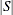, then the number of blocks is 1, and the number of copies of original variables is 0. Using one block would be equivalent to the original staircase formulation and would not yield a model conducive to decomposition. As the number of blocks is increased, the number of linking variables increases (the size of the master problem), the strength of the decomposition bound decreases, and the difficulty of solving the subproblems decreases. In addition, as the number of blocks and their relative difficulty change, the efficient utilization of your machine’s parallel architecture can be affected.

The previous section used a block size of 40. The following statement calls the decomposition algorithm and uses a block size of 130:

%ResourceAllocation_Decomp(task_data=TaskData, capacity=100, block_size=130);


The solution summary is displayed in Output 13.5.7.

Output 13.5.7: Solution Summary

The OPTMODEL Procedure

Solution Summary
Solver MILP
Algorithm Decomposition
Objective Function TotalProfit
Solution Status Conditionally Optimal
Objective Value 62524.000312

Relative Gap 0.0000746713
Absolute Gap 4.6690962869
Primal Infeasibility 9.5560876E-6
Bound Infeasibility 1.776357E-15
Integer Infeasibility 9.5560876E-6

Best Bound 62528.669409
Nodes 1
Iterations 22
Presolve Time 2.60
Solution Time 27.04

The iteration log, which contains the problem statistics, the progress of the solution, and the optimal objective value, is shown in Output 13.5.8.

Output 13.5.8: Log

 NOTE: There were 2697 observations read from the data set WORK.TASKDATA. NOTE: Problem generation will use 4 threads. NOTE: The problem has 2877 variables (0 free, 0 fixed). NOTE: The problem has 2877 binary and 0 integer variables. NOTE: The problem has 2868 linear constraints (2688 LE, 180 EQ, 0 GE, 0 range). NOTE: The problem has 27240 linear constraint coefficients. NOTE: The problem has 0 nonlinear constraints (0 LE, 0 EQ, 0 GE, 0 range). NOTE: The MILP presolver value BASIC is applied. NOTE: The MILP presolver removed 0 variables and 0 constraints. NOTE: The MILP presolver removed 0 constraint coefficients. NOTE: The MILP presolver modified 0 constraint coefficients. NOTE: The presolved problem has 2877 variables, 2868 constraints, and 27240 constraint coefficients. NOTE: The MILP solver is called. NOTE: The Decomposition algorithm is used. NOTE: The Decomposition algorithm is executing in single-machine mode. NOTE: The DECOMP method value USER is applied. NOTE: The decomposition subproblems consist of 21 disjoint blocks. NOTE: The decomposition subproblems cover 2877 (100.00%) variables and 2688 (93.72%) constraints. NOTE: The deterministic parallel mode is enabled. NOTE: The Decomposition algorithm is using up to 4 threads. Iter         Best       Master         Best       LP       IP   CPU  Real Bound    Objective      Integer      Gap      Gap  Time  Time NOTE: Starting phase 1. 1       0.0000       0.0000            .    0.00%        .     2     0 NOTE: Starting phase 2. .   63337.0002   51369.0000   51369.0000   18.90%   18.90%     2     0 3   63337.0002   51989.0000   51989.0000   17.92%   17.92%     8     2 10   63337.0002   61546.0016   51989.0000    2.83%   17.92%    29    10 .   63337.0002   61824.1123   60178.9998    2.39%    4.99%    29    10 13   63124.2360   62171.2306   60178.9998    1.51%    4.67%    38    13 14   63106.9999   62354.0001   60178.9998    1.19%    4.64%    40    14 15   62791.3011   62392.5003   60178.9998    0.64%    4.16%    43    15 16   62676.0007   62491.0002   60178.9998    0.30%    3.98%    46    16 17   62596.0020   62524.0003   62524.0003    0.12%    0.12%    49    17 18   62568.5024   62524.0003   62524.0003    0.07%    0.07%    52    18 19   62568.5024   62524.0003   62524.0003    0.07%    0.07%    54    19 20   62531.0027   62524.0003   62524.0003    0.01%    0.01%    57    20 .   62531.0027   62524.0003   62524.0003    0.01%    0.01%    57    20 22   62528.6694   62524.0003   62524.0003    0.01%    0.01%    63    22 NOTE: The Decomposition algorithm stopped on the integer RELOBJGAP= option. Node  Active   Sols         Best         Best      Gap     CPU    Real Integer        Bound             Time    Time 0       0     11   62524.0003   62528.6694    0.01%      63      22 NOTE: The Decomposition algorithm used 4 threads. NOTE: The Decomposition algorithm time is 22.57 seconds. NOTE: Conditional optimal. NOTE: Objective = 62524.000312.

This version of the model provides a stronger bound and yields better overall performance.